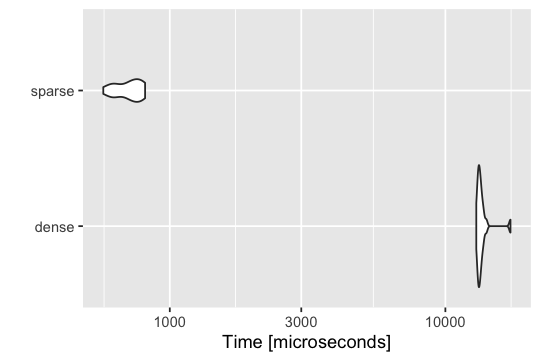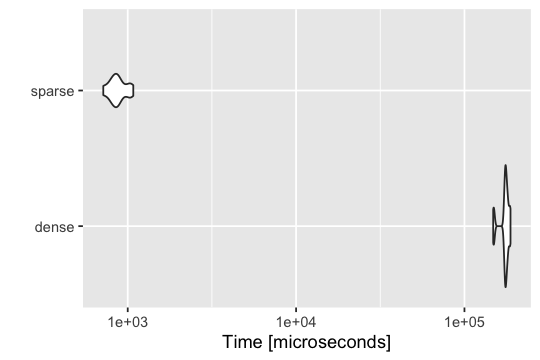# Sparse matrix multiplication strategy

## Set up environment

library(susieR)
library(Matrix)
library(microbenchmark)
library(ggplot2)
set.seed(1)

## Goal

Our intention is to use sparse matrix multiplications to help reduce computation time.

## General strategy

Given a large sparse matrix X, we want to compute some matrix multiplications associated with a scaled $$\tilde{X}$$. We notice that after scaling, X becomes a dense matrix and is not possible for a sparse matrix multiplication. So we construct formulae to apply sparse matrix multiplication first on a standardized X since standardization does not affect its sparsity. Then we perform centering to get the same result.

## Types of matrix multiplications

There are two types of matrix multiplications we want to investigate:

• Compute $$\tilde{X}b$$, where $$\tilde{X}$$ is an n by p scaled matrix and $$b$$ is a p vector.

• Compute $$\tilde{X}^Ty$$, where $$\tilde{X}$$ is an n by p scaled matrix and $$y$$ is an n vector.

## Results

This strategy has a decent performance when computing both $$\tilde{X}b$$ and $$\tilde{X}^Ty$$, compared to simple matrix multiplication %*%.

## Strategy formulae details

### Computing $$\boldsymbol{\tilde{X}b}$$

Suppose we want to compute $$\tilde{X}b$$, where $$\tilde{X}$$ is a scaled n by p matrix and $$b$$ is a p vector. Our goal is to express $$\tilde{X}b$$ into a term involving unscaled $$X$$ matrix multiplication to achieve sparse matrix operation.

\begin{equation} \begin{aligned} \tilde{X}b &= \sum_{j=1}^{p} \tilde{X}_{\cdot j} b_j \\ &= \sum_{j=1}^{p} \frac{X_{\cdot j}-\mu_j}{\sigma_j}b_j \\ &= \sum_{j=1}^{p}\frac{X_{\cdot j}}{\sigma_j}b_j - \sum_{j=1}^{p} \frac{\mu_j}{\sigma_j}b_j \\ &= X b / \sigma - \mu^Tb/\sigma, \end{aligned} \end{equation}

where $$\mu$$ is a p-vector of column means, and $$\sigma$$ is a p-vector of column standard deviations.

### Computing $$\boldsymbol{\tilde{X}^Ty}$$

Suppose we want to compute $$\tilde{X}^Ty$$, where $$\tilde{X}$$ is a scaled n by p matrix and $$y$$ is an n vector. Similarly, we express $$\tilde{X}^Ty$$ using unscaled $$X$$ so that we can perform sparse matrix multiplication. We have the following:

\begin{equation} \begin{aligned} \tilde{X}^Ty &= \sum_{i=1}^{n} \tilde{X}_{i.}y_i \\ &= \sum_{i=1}^{n} \frac{X_{i.} - \mu}{\sigma}y_i \\ &= \frac{1}{\sigma}\sum_{i=1}^{n}X_{i.}y_i - \frac{\mu}{\sigma}\sum_{i=1}^{n} y_i \\ &= \frac{1}{\sigma}(X^Ty) - \frac{\mu}{\sigma}y^T 1, \end{aligned} \end{equation}

where $$\mu$$ is a p-vector of column means, and $$\sigma$$ is a p-vector of columnwise standard deviations.

## Simulations

We simulate an n = 1000 by p = 10000 matrix X at sparsity $$99\%$$, i.e. $$99\%$$ entries are zeros. We compare results between normal matrix computation and our sparse strategy as well as comparing speed using microbenchmark.

create_sparsity_mat <- function(sparsity, n, p) {
nonzero          <- round(n*p*(1-sparsity))
nonzero.idx      <- sample(n*p, nonzero)
mat              <- numeric(n*p)
mat[nonzero.idx] <- 1
mat              <- matrix(mat, nrow=n,ncol=p)
return(mat)
}
n <- 1000
p <- 10000
X.dense  <- create_sparsity_mat(0.99,n,p)
X.sparse <- as(X.dense,"dgCMatrix")
X.tilde  <- susieR:::set_X_attributes(X.dense) #returns a scaled X if input is a dense matrix
X <- susieR:::set_X_attributes(X.sparse) #return an unsacled sparse X if input is a sparse matrix
#but computes column means and standard deviations
b <- rnorm(p)
y <- rnorm(n)

### Benchmark for computing $$\boldsymbol{\tilde{X}b}$$

The final results of two methods when computing $$\tilde{X}b$$ are very close.

res1 <- X.tilde %*% b
res2 <- susieR:::compute_Xb(X,b)
max(abs(res1 - res2))
#  362.79
compute_Xb_benchmark <- microbenchmark(
dense  = (use.normal.Xb <- X.tilde%*%b),
sparse = (use.sparse.Xb <- susieR:::compute_Xb(X,b)),
times = 20,unit = "s")

Our sparse strategy demonstrates an obvious advantage over the normal matrix multiplication in computing $$\tilde{X}b$$.

autoplot(compute_Xb_benchmark)
# Coordinate system already present. Adding new coordinate system, which will replace the existing one.### Benchmark for computing $$\boldsymbol{\tilde{X}^Ty}$$

The final results of two methods when computing $$\tilde{X}^Ty$$ are almost the same.

res3 <- t(X.tilde) %*% y
res4 <- susieR:::compute_Xty(X,y)
max(abs(res3 - res4))
#  119.2975
compute_Xty_benchmark = microbenchmark(
dense  = (use.normal.Xty <- t(X.tilde)%*%y),
sparse = (use.sparse.Xty <- susieR:::compute_Xty(X, y)),
times = 20,unit = "s")

Our sparse strategy evidently has a better performance than the normal method in computing $$\tilde{X}^Ty$$.

autoplot(compute_Xty_benchmark)
# Coordinate system already present. Adding new coordinate system, which will replace the existing one.